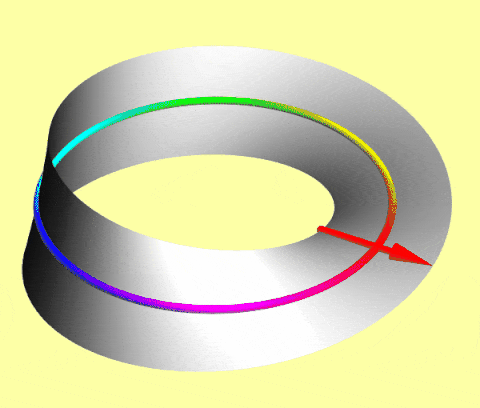# Spinors

Fermions like electrons, protons, and neutrons inhabit a 720° world: 360° rotations negate their quantum states, but 720° rotations restore them.

A simple macroscopic model of such spinors is an arrow translating on a Möbius strip: as the center circle rotates, the attached arrow flips after 360° but flips back after 720°.As the circle rotates, the attached arrow flips after 360° and flips back after 720°

In Dirac notation

$$R_{2\pi}|\psi \rangle = -|\psi \rangle = e^{i \pi}|\psi \rangle,$$

but

$$R_{4\pi}|\psi \rangle = +|\psi \rangle= e^{i 2\pi}|\psi \rangle,$$

where the ket $|\psi\rangle$ is the state, the exponentials are phase factors, and their arguments are phase shifts.

To detect a relative phase shift, send a neutron via two paths, rotate it along one path with a magnetic field (coupled to its magnetic dipole moment), and observe destructive interference for 360° rotations and constructive interference for 720° rotations. (The experiment is harder with charged electrons and protons, whose translation is deflected by the magnetic field.)